Next: Using QFDFT to obtain Up: Density functional theory Previous: Density functional theory

### QFDFT: A formalism of time dependent DFT

Quantum Fluid Dynamics and Quantum Fluid Density Functional Theory

Schrödinger's formulation of quantum mechanics in terms of the complex wave function did not have any direct physical significance as was possible in classical mechanics. This inherent shortcoming inspired workers to seek for explanations for quantum phenomena within the framework of classical mechanics. The hydrodynamic interpretation of quantum mechanics by Madelung, better known as quantum fluid dynamics (QFD), is one of the earliest such attempts. In QFD, the wave function for a particle is expressed in polar form asIts real and imaginary parts are then separated, whereby the time-dependent Schrödinger equation for a single particle is transformed to two fluid-dynamical equations, viz., the continuity equation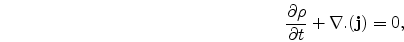and an Euler-type equation of motion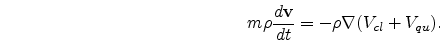Here m is the mass of the particle.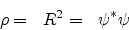is the single-particle density.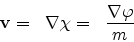is the velocity,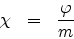being the velocity potential.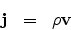is the current density.

Vcl is the classical potential, andis the quantum potential.

Thus we observe that in QFD the time-evolution of a system can be studied by visualizing the system as a flowing probability fluid of density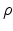(r, t) and having a local' velocity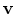(r, t) or a local' current density j(r, t). It is observed that the quantum fluid evolves not only due to the classical Coulomb potential Vcl but also due to an additional potential Vqu, which is the quantum potential of hidden variable theory . The time evolution of the quantum system can therefore be studied through the solution of the two QFD equations to obtain(r, t) and j(r, t). The initial values(r, 0) and j(r, 0) must be prescribed for the purpose. The QFD equations can be written as quantum analogues of classical Hamilton's equations of motion, when the classical Hamiltonian is replaced by a properly chosen energy functional of DFT and one considersand (-) as the canonically conjugate variables. In the remaining part of this section as also in the following chapters, we would be considering atomic units (m = 1 and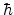= 1). The QFD equations for a given potential can be derived from the following quantum Hamilton's equations",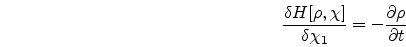and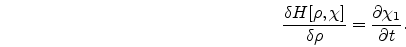In the above equations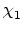is (-) and the Hamiltonian functional[,] is given bywhere the first term is the macroscopic kinetic energy (KE), second term refers to the intrinsic KE whose functional form was first suggested by von Weizsäcker [] and the last term is the potential energy. These equations can also be obtained from a least action principle [153-156].          The variational equation may be written in this case aswhere the Lagrangian functional is given in terms of the above Hamiltonian functional asInitially, Madelung's formalism did not have many takers. The renewed interest in QFD in the last few decades is attributed to the work of Bohm and Takabayashi. Since then QFD has found many applications in atomic, molecular, solid state and nuclear physics .

Extension of the QFD approach to many-particle systems is not straightforward. However, within the Hartree theory, Hartree-Fock theory and in terms of natural orbitals such an extension has been accomplished. Furthermore, the formal equivalence of QFD and the time-dependent density functional theory (TDDFT) has been established. An amalgamation of TDDFT and QFD gives rise to a quantum fluid density functional theory (QFDFT) in which an N-electron system is mapped onto a system of N noninteracting particles moving under the influence of a nonlinear one-body effective potential , Veff(r, t).

The central equation of QFDFT is the following generalized nonlinear Schrdinger equation(GNLSE)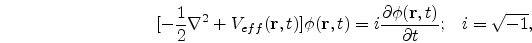obtained by combining the QFD equations for many-particle systems and making use of various time-dependent density functionals. The explicit form for Veff(r, t) depends on that of the Hohenberg-Kohn functional F[]. The charge and current densities can be obtained as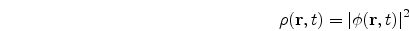and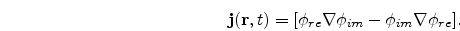One may note that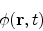is not the conventional wavefunction unless the system of interest is a single particle one. Time evolution of any dynamical quantity can be monitored by making use of the charge and current densities at a given time as obtained from the last two equations.Next: Using QFDFT to obtain Up: Density functional theory Previous: Density functional theory
Abhijit Poddar
2007-09-27